# High School Chemistry : Help with Isotopes and Ions

## Example Questions

← Previous 1

### Example Question #1 : Help With Isotopes And Ions

Which of the following is a naturally occurring isotope of carbon-12?

Carbon-13

Carbon-6

Carbon-9

Carbon-8

Carbon-7

Carbon-13

Explanation:

Carbon is an element with six protons (atomic number six), and most commonly has six neutrons. This brings the total mass of carbon to twelve atomic mass units (12amu). There are isotopes of carbon with seven or eight neutrons, which are radioactive. These isotopes correspond to carbon-13 and carbon-14. The true atomic mass of carbon on the periodic table is 12.011amu, accounting for small contributions by these heavier isotopes.

Carbon-6, cabron-7, carbon-8, and carbon-9 imply isotopes with zero, one, two, and three neutrons respectively. Though these isotopes could theoretically exist, they would be extremely unstable and do not occur in nature.

### Example Question #1 : Help With Isotopes And Ions

If a neutron is added to the nucleus of an atom, which of the following has changed?

The atomic number

The charge

The element identity

The mass number

The mass number

Explanation:

Isotopes are variations of an element that differ by the number of neutrons in the nucleus. Neutrons are neutrally charged, so adding or removing them from a nucleus does not alter the charge of the atom. The atomic number is the number of protons in an atom, and the mass number is the sum of both protons and neutrons in the nucleus. As a result, the mass number will change by adding a neutron.

The number of protons is equal to the atomic number of the element. Adding or subtracting protons will change the element's identity. Ions can be created by changing the number of electrons and isotopes can be created by changing the number neutrons, but changing the number of protons changes the element.

### Example Question #1 : Help With Isotopes And Ions

Which of the following will result in an ion?

The atomic number changes

The number of protons changes

The number of neutrons changes

The number of electrons changes

The number of electrons changes

Explanation:

Ions are atoms that have gained or lost electrons. This results in an atom with a charge. Atoms with a neutral charge have an equal number of protons and electrons. Changing the electron number will result in a charge on the atom.

For example, adding an electron to chlorine creates a chlorine anion.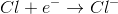### Example Question #31 : Elements And Atoms

Which of the following elements is isoelectronic with an isotope of fluorine?

Fluorine

Oxygen

Sulfur

Nitrogen

Neon

Fluorine

Explanation:

Two atoms are isoelectric if they each have the same number of electrons. Ions of an element will vary in the number of electrons, but isotopes will vary in the number of neutrons only. In its ground state, fluorine has 9 electrons, equal to the number of protons in order to have a neutral atom. Any ground state isotopes of fluorine will also have 9 electrons, making them isoelectric.

### Example Question #5 : Help With Isotopes And Ions

Which of the following elements is isoelectronic with an oxygen ion?

Selenium

Neon

Sulfur

Fluorine

Nitrogen

Neon

Explanation:

Two atoms are isoelectric if they each have the same number of electrons. In its ground state, oxygen has 8 electrons, equal to the number of protons in order to have a neutral atom. In order to become a stable ion, the oxygen atom must gain two electrons. This gives it a charge of negative two, but allows it to satisfy the octet rule, making it a very stable ion. With the ionization, the atom now has 10 electrons.

Neon has atomic number 10, meaning that it will also have 10 electrons in its ground state.

### Example Question #1 : Help With Isotopes And Ions

Which element is isoelectronic with a bromide ion?

Iodine

None of these

Krypton

Selenium

Chlorine

Krypton

Explanation:

Two atoms are isoelectric if they each have the same number of electrons. In its ground state, bromine has 35 electrons, equal to the number of protons in order to have a neutral atom. In order to become a bromide ion, the bromine atom must gain one electron. This gives it a charge of negative one, but allows it to satisfy the octet rule, making it a very stable ion. With the ionization, the atom now has 36 electrons.

Krypton has atomic number 36, meaning that it will also have 36 electrons in its ground state.

### Example Question #7 : Help With Isotopes And Ions

Which of the following answer choices is not isoelectronic with the other atoms listed?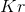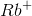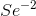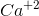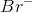Explanation:

Isoelectronic atoms are atoms or ions that contain the same number of total electrons.is not isoelectronic with the rest of the ions and atoms listed because it contains a total of ten electrons, while the rest in the series contain thirty-six.

Positively charged ions (cations) are formed when electrons are removed from the outer shell of the atom. Removing a negatively charged electron gives the ion a positive charge. For example, the element rubidium,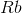, in its ground state contains thirty-seven electrons. The ioncontains one less, thirty-six, making it isoelectronic with krypton and the other ions and atoms in the series.

Negatively charge ions (anions) are formed when electrons are added to the outer shell of the atom. Adding a negatively charged electron gives the ion a negative charge. For example, ground state selenium,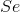, contains thirty-four electrons. The ion, has two added electrons giving it a total of thirty-six electrons. The ion is isoelectronic with the other ions and atoms in the series.

### Example Question #1 : Help With Isotopes And Ions

Which is the isotopic notation for an isotope of an element with 19 protons and 20 neutrons.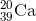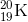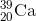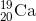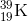Explanation:

The general isotopic notation is as follows: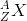, whereis the mass number, which is equal to the number of neutrons plus protons, and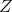is the atomic number, which is equal to the number of protons. We know that the atomic number determines the identity of an element, thus an atom with 19 protons must be potassium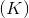. Following the isotopic notation mentioned above, our mass number is 39.

### Example Question #1 : Help With Isotopes And Ions

What is the isotopic notation for an isotope of an element with a mass number of 197 and 118 neutrons?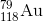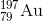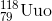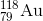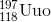Explanation:

The general isotopic notation is as follows:, whereis the mass number, which is equal to the number of neutrons plus protons, andis the atomic number, which is equal to the number of protons. We know that the atomic number determines the identity of an element, thus an atom with a mass number of 197 and 118 neutrons must have 79 protons. Thus the element is gold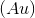.

### Example Question #10 : Help With Isotopes And Ions

Identify the number of protons, neutrons, and electrons in a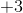charged cation of aluminum (mass number = 27).

Protons=13

Neutrons=14

Electrons=13

Protons=10

Neutrons=14

Electrons=16

Protons=10

Neutrons=14

Electrons=13

Protons=16

Neutrons=14

Electrons=13

Protons=13

Neutrons=14

Electrons=10

Protons=13

Neutrons=14

Electrons=10

Explanation:

The atomic number of aluminum is 13, which means it has 13 protons. All atoms with 13 protons are called aluminum. The mass number of aluminum is 27 and is the sum of neutrons and protons. We simply subtract the number of protons from the mass number to get the number of neutrons. Normally there are an equal number of electrons and protons. But since this aluminum exists as an ion with a +3 charge, it has lost three electrons and has only 10 electrons.

← Previous 1

### All High School Chemistry Resources